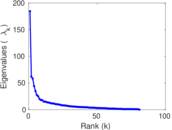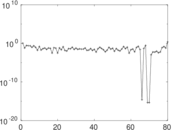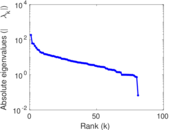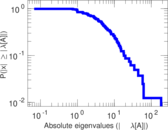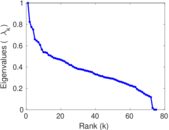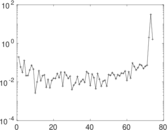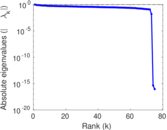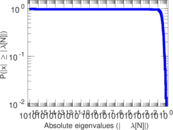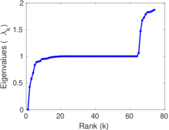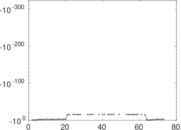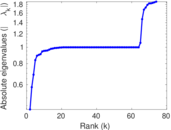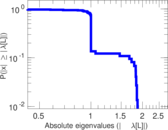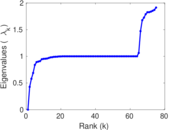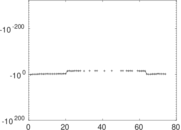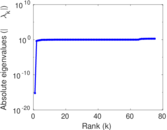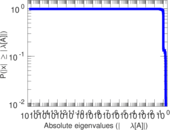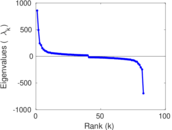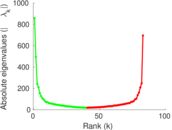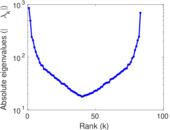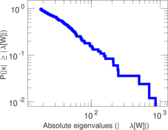# Wikipedia edits (atj)

This is the bipartite edit network of the Atikamekw Wikipedia. It contains users and pages from the Atikamekw Wikipedia, connected by edit events. Each edge represents an edit. The dataset includes the timestamp of each edit.

 Code `atj` Internal name `edit-atjwiki` Name Wikipedia edits (atj) Data source http://dumps.wikimedia.org/ AvailabilityDataset is available for download Consistency checkDataset passed all tests Category Authorship network Dataset timestamp 2017-10-20 Node meaning User, article Edge meaning Edit Network formatBipartite, undirected Edge typeUnweighted, multiple edges Temporal dataEdges are annotated with timestamps

## Statistics

 Size n = 655 Left size n1 = 83 Right size n2 = 572 Volume m = 6,306 Unique edge count m̿ = 3,266 Wedge count s = 459,277 Claw count z = 58,368,889 Cross count x = 6,181,474,537 Square count q = 772,689 4-Tour count T4 = 8,030,876 Maximum degree dmax = 1,839 Maximum left degree d1max = 1,839 Maximum right degree d2max = 225 Average degree d = 19.255 0 Average left degree d1 = 75.975 9 Average right degree d2 = 11.024 5 Fill p = 0.068 792 7 Average edge multiplicity m̃ = 1.930 80 Size of LCC N = 632 Diameter δ = 6 50-Percentile effective diameter δ0.5 = 1.678 76 90-Percentile effective diameter δ0.9 = 2.912 90 Median distance δM = 2 Mean distance δm = 2.338 84 Gini coefficient G = 0.679 733 Balanced inequality ratio P = 0.250 951 Left balanced inequality ratio P1 = 0.148 906 Right balanced inequality ratio P2 = 0.341 262 Relative edge distribution entropy Her = 0.809 390 Power law exponent γ = 1.650 41 Tail power law exponent γt = 2.551 00 Tail power law exponent with p γ3 = 2.551 00 p-value p = 0.000 00 Left tail power law exponent with p γ3,1 = 1.731 00 Left p-value p1 = 0.516 000 Right tail power law exponent with p γ3,2 = 8.331 00 Right p-value p2 = 0.017 000 0 Degree assortativity ρ = −0.292 854 Degree assortativity p-value pρ = 1.332 26 × 10−65 Spectral norm α = 184.526 Algebraic connectivity a = 0.426 775 Spectral separation |λ1[A] / λ2[A]| = 2.980 76 Controllability C = 491 Relative controllability Cr = 0.751 914

## Plots

### Fruchterman–Reingold graph drawing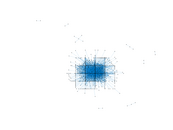### Degree distribution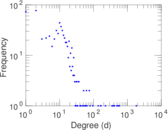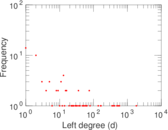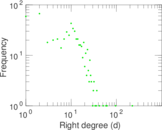### Cumulative degree distribution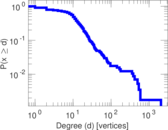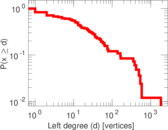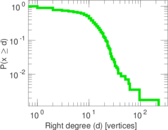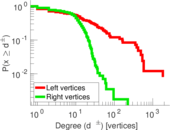### Lorenz curve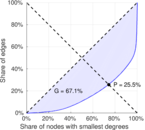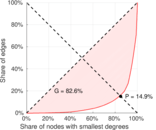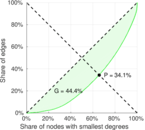### Spectral distribution of the adjacency matrix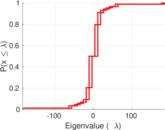### Spectral distribution of the normalized adjacency matrix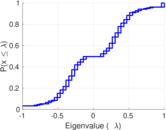### Spectral distribution of the Laplacian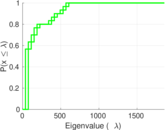### Spectral graph drawing based on the adjacency matrix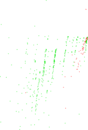### Spectral graph drawing based on the Laplacian### Spectral graph drawing based on the normalized adjacency matrix### Degree assortativity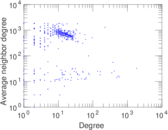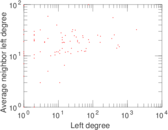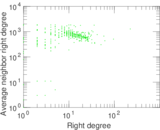### Zipf plot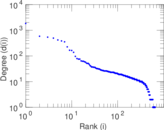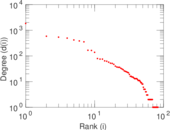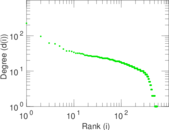### Hop distribution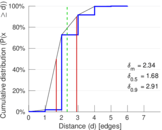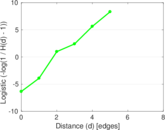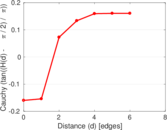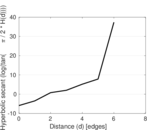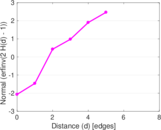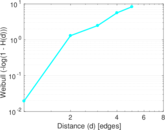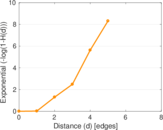### Double Laplacian graph drawing### Delaunay graph drawing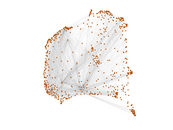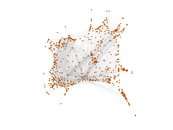### Edge weight/multiplicity distribution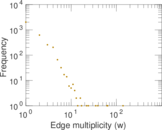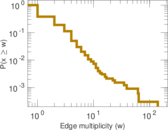### Temporal distribution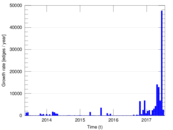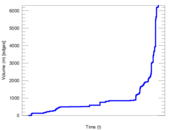### Temporal hop distribution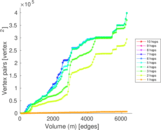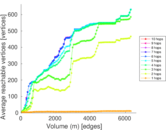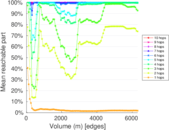### Diameter/density evolution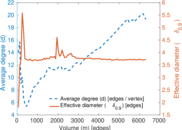### Matrix decompositions plots